Maths-
General
Easy

Question

# 8 Arrange the following rational numbers in ascending order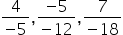and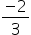.Hint:

## The correct answer is: Yes it's rational numbers

### Given rational numbers areand.Express the rational numbers with positive denominator by multiplying with -1.Here,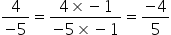and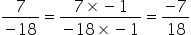So, Now we have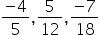and.By taking LCM of denominators 5,12,18 and 3, we get LCM as 180On multiplying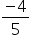with 36, we get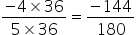On multiplying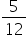with 15, we get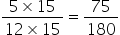On multiplying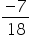with 10, we get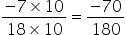On multiplyingwith 60, we get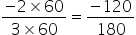On comparing the above fractions with same denominator,we have -144 < -120< -70 < 75Hence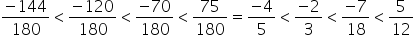So ascending order is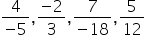#### With Turito Foundation.#### Get an Expert Advice From Turito.